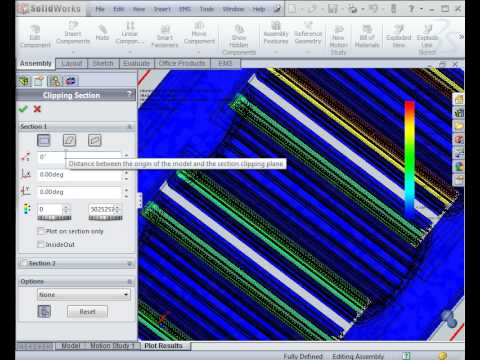FULLY EMBEDDED SIMULATION

# 3-phase cylindrical transformer

HOME / Applications / 3-phase cylindrical transformer

## 3 phase cylindrical transformer

Transformers are used to convert a higher voltage into a lower, safer voltage (or vice versa) that appliances and other electrical devices can use. The voltage is sent across power lines at a higher voltage to limit power loss across the grid. Transformers can either increase or decrease voltage without modifying the current's frequency or the amount of power being transferred between windings via a magnetic circuit. Three-phase circuits are the most economical for AC power transmission and distribution.

## Solid works model of a 3 phase cylindrical transformer

The Solid works model of a 3 Phase cylindrical transformer consists of a Core, three primary coils and three secondary coils, as shown in Figure 1.Figure 1 - 3D model of a 3 phase cylindrical transformer

## Open circuit simulation of a 3 phase cylindrical transformer in EMS

In order to calculate the core loss, an open circuit simulation of a 3-phase cylindrical transformer, is carried out using AC Magnetic study coupled with thermal analysis.

## Coupling to Thermal Analysis

In EMS, Thermal analysis automatically follow an electromagnetic analysis so that the heat sources in the model are automatically pre-computed. Thermal analysis calculates the temperature distribution in solids due to conduction. Figure 3 illustrates how to couple AC Magnetic study to Steady state Thermal analysis.

## Material

The simulated model is composed of laminated steel Core, three primary coils, three secondary coils and Air. The properties of the materials are summarized in Table 1.
The core loss curve of M36 is shown in Figure 2.

Table 1 - Materials used in the EMS simulation

 Component Material Relative permeability Electrical Conductivity (S/m) Thermal Conductivity (W*m-1  * k-1) Core laminated steel (M36 at 0.36mm; Mass density: 7700 kg/m^3) 1616 2.32558 e+006 43 Primary Coils/Secondary Coils Copper 0.99991 5.7e+007 401 Coils Air, Inner Air, Outer Air Air 1 0 0.024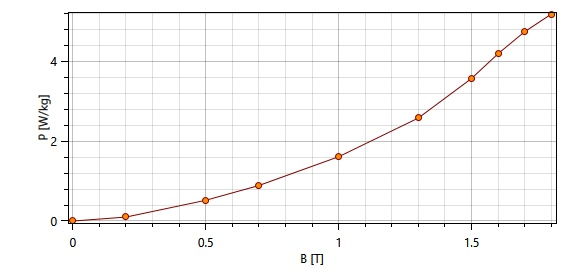Figure 2 - Core BP Curve

## Coils definition in an open circuit simulation

Open circuit operation of a 3-phase transformer is performed by applying the rated voltage to the primary windings and keeping the secondary windings open. Since no load is connected across the secondary windings, the three coils of the secondary of the transformer are modeled as solid coils, with 0 Ampere as Net RMS current, while the three primary coils are modeled as wound coils passing 250 A-turns (50 turns, 5A) and each one differs from the other by 120 time-degrees in phase.  The coils wire diameter, for both primary and secondary coils, was set to be 19 AWG.

## Thermal Inputs

Convection is the heat transfer mode in which heat transfers between a solid face and an adjacent moving fluid (or gas). In EMS, all Air components are given a convection boundary condition.

## Simulation Results

In AC Magnetic study, EMS compute inductance, resistance, impedance, coupling coefficient, current, induced voltage, flux linkage, leakage inductance, current, induced voltage, winding loss, core loss and energy.
Eddy loss, Hysteresis loss, Excess loss and Core loss in the core of the transformer are computed by EMS in open circuit simulation, and shown in Figure 3.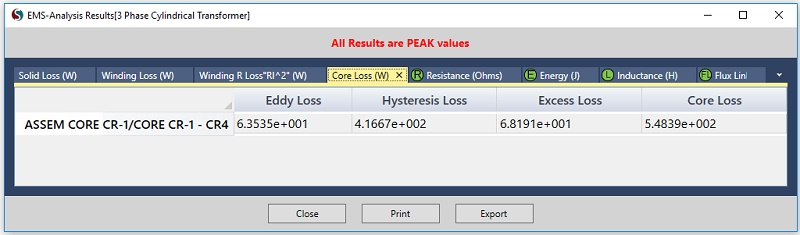Figure 3 - Results table in EMS

## 3D fields generated by AC Magnetic study coupled to Thermal Analysis

The Magnetic Flux Density in the transformer, the Magnetic Field Intensity, the Core Loss, the Temperature, and the Heat Flux 3D plots generated by EMS in open circuit simulation are given in the figures 4, 5, 6, 7 and 8 respectively.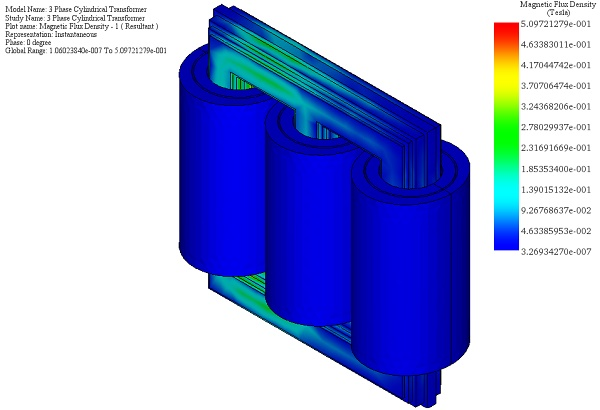Figure 4 - Magnetic flux density in the transformer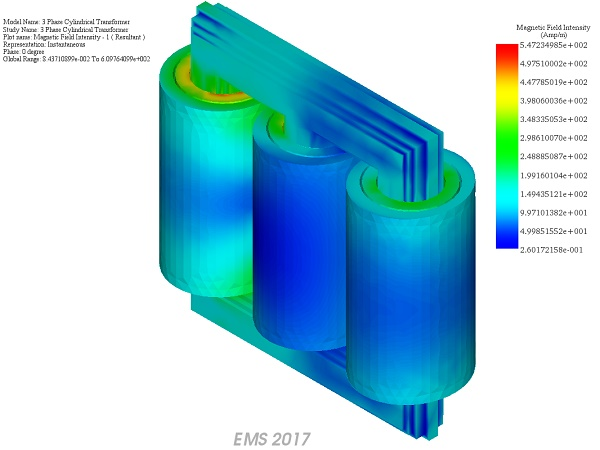Figure 5 - Magnetic flux intensity in the transformer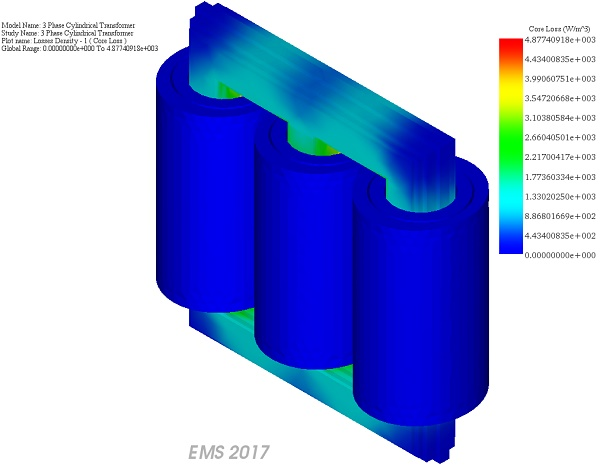Figure 6 - Core Loss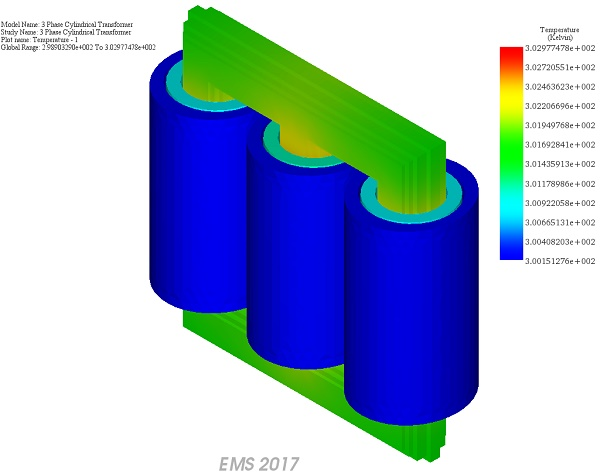Figure 7 - Temperature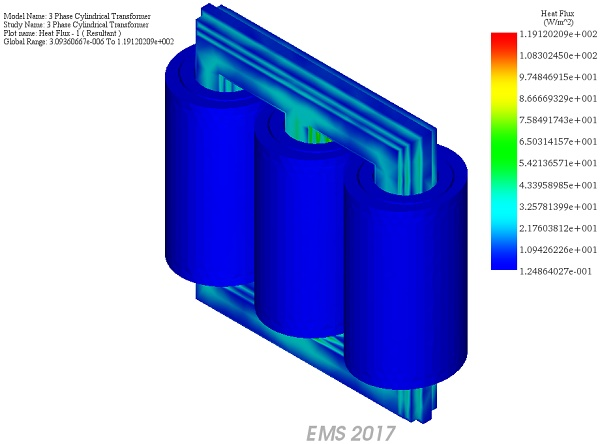Figure 8 - Heat flux

## Conclusion

In this article, an electromagnetic study coupled with steady state thermal analysis of a three phase cylindrical transformer model is carried out. The applied current density in each coil, the magnetic flux density, the magnetic field intensity, the applied current density, the resulting eddy current distribution, winding loss, Temperature, Heat flux and other electromagnetic parameters calculated by EMS are presented.

### Videos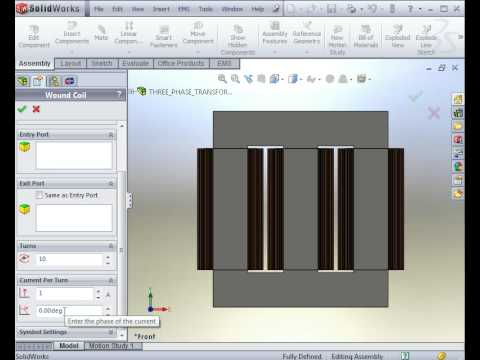#### AC Magnetic Analysis of a Transformer 1/2